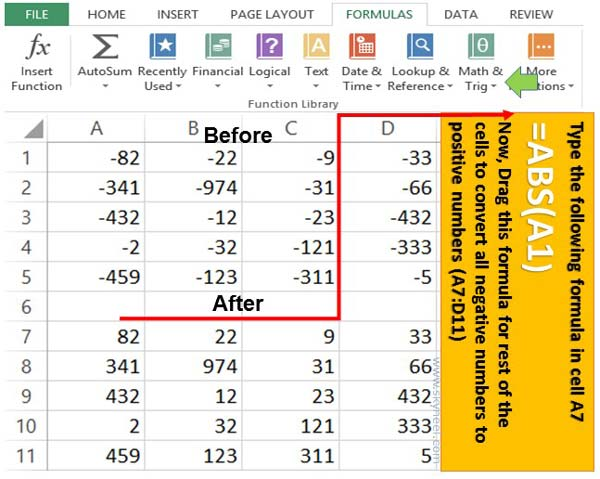# Change negative numbers to positive numbers – ABS function

ABS is an important Math & Trig Excel function which is used to change negative numbers to positive numbers. ABS stands for Absolute value.

During working on the MS Excel, sometime we need to convert or change negative values to positive. If you have large worksheet and do this job by manually it will be difficult. Now, today we discuss about Excel ABS function change negative numbers to positive numbers. MS Excel is very popular and interesting electronic spreadsheet software. There are billion of users are working on this application software around the world.

There are lot of tips and tricks available for MS Excel. These tips and tricks help different users and solve different problems which they face during the working on Excel.  With the help of ABS function you can easily convert any negative number into positive number in MS Excel. You can also easily convert positive numbers to negative numbers in MS Excel.

## Excel ABS function- Change negative numbers to positive numbers

Sometime when you apply any formula to get the result, most of the values are converted into negative values. If you want to convert all negative numbers to positive numbers manually, it will take lot of time. Now, Excel ABS function allows you to change -ve numbers to +ve numbers on quick basis. You just have to take few simple given steps to convert all negative numbers to positive numbers.

### ABS Function in MS Excel

ABS is an important function which is used to return absolute value of a given number, a number without a sign. In other words, this ABS function is used to convert negative numbers to positive numbers. ABS stands for Absolute. The ABS function is found in the Math & Trig category of Excel functions.

### Syntax of ABS Function:

=ABS(number)

number: It is the real number for which you want the absolute value.Step 1: Open an existing worksheet in which you have lot of negative number which you want to convert them in to positive numbers.

Step 2: Now take cursor on new location any type the following given ABS function to convert negative numbers in to positive numbers. For eg. Take cursor on cell A7 and type the given formula  =ABS(A1) to change to negative value of cell A1. Now you can check negative number converted into positive number.

Step 3: Drag this formula for rest of the cells to convert all the negative numbers into positive numbers.

Excel ABS function change -ve numbers to +ve numbers article help all those guys who are facing this problem too much. If you have any query regarding this article please write us in the comment box. Thanks to all.